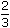# Aptitude - Races and Games

Exercise : Races and Games - General Questions
11.
In a 300 m race A beats B by 22.5 m or 6 seconds. B's time over the course is:
86 sec
80 sec
76 sec
None of these
Explanation:

 B runs 45 m in 6 sec. 2B covers 300 m in6 x 2 x 300sec = 80 sec. 45

12.
A runs 1times as fast as B. If A gives B a start of 80 m, how far must the winning post be so that A and B might reach it at the same time?
200 m
300 m
270 m
160 m
Explanation:

 Ratio of the speeds of A and B = 5 : 1 = 5 : 3. 3

Thus, in race of 5 m, A gains 2 m over B.

2 m are gained by A in a race of 5 m.

 80 m will be gained by A in race of5 x 80m = 200 m. 2Winning post is 200 m away from the starting point.

13.
In a 100 m race, A can beat B by 25 m and B can beat C by 4 m. In the same race, A can beat C by:
21 m
26 m
28 m
29 mA : C =A x B=100 x 100= 100 = 100 : 72. B C 75 96 72A beats C by (100 - 72) m = 28 m.